In Progress
Lesson 12 of 24
In Progress

# Continuity

Many functions have the property that their graphs can be traced with a pencil without lifting the pencil from the page. Such functions are called continuous. Other functions have points at which a break in the graph occurs, but satisfy this property over intervals contained in their domains. They are continuous on these intervals and are said to have a discontinuity at a point where a break occurs.

We begin our investigation of continuity by exploring what it means for a function to have continuity at a point. Intuitively, a function is continuous at a particular point if there is no break in its graph at that point.

# Continuity at a Point

Before we look at a formal definition of what it means for a function to be continuous at a point, let’s consider various functions that fail to meet our intuitive notion of what it means to be continuous at a point. We then create a list of conditions that prevent such failures.

Our first function of interest is shown in (Figure). We see that the graph ofhas a hole at. In fact,is undefined. At the very least, forto be continuous at, we need the following conditions:i.is defined.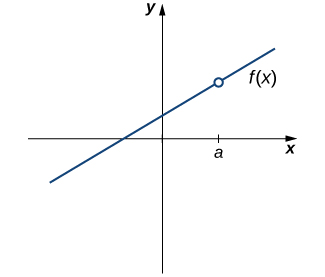Figure 1. The function  is not continuous at a because  is undefined.

However, as we see in (Figure), this condition alone is insufficient to guarantee continuity at the point. Althoughis defined, the function has a gap at. In this example, the gap exists becausedoes not exist. We must add another condition for continuity at—namely,ii.exists.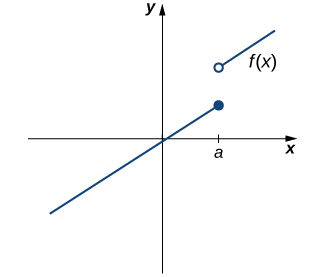Figure 2. The function  is not continuous at a because  does not exist.

However, as we see in (Figure), these two conditions by themselves do not guarantee continuity at a point. The function in this figure satisfies both of our first two conditions, but is still not continuous at. We must add a third condition to our list:iii..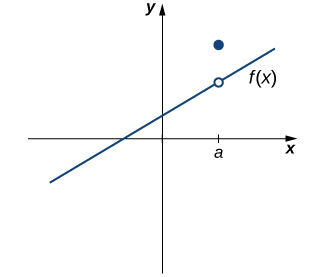Figure 3. The function  is not continuous at a because .

Now we put our list of conditions together and form a definition of continuity at a point.

The next three examples demonstrate how to apply this definition to determine whether a function is continuous at a given point. These examples illustrate situations in which each of the conditions for continuity in the definition succeed or fail.

### Determining Continuity at a Point, Condition 2

Using the definition, determine whether the function. Justify the conclusion.

#### Solution

Let’s begin by trying to calculate..

Thus,is defined. Next, we calculate. To do this, we must computeand:

and.

Therefore,does not exist. Thus,is not continuous at 3. The graph ofis shown in (Figure).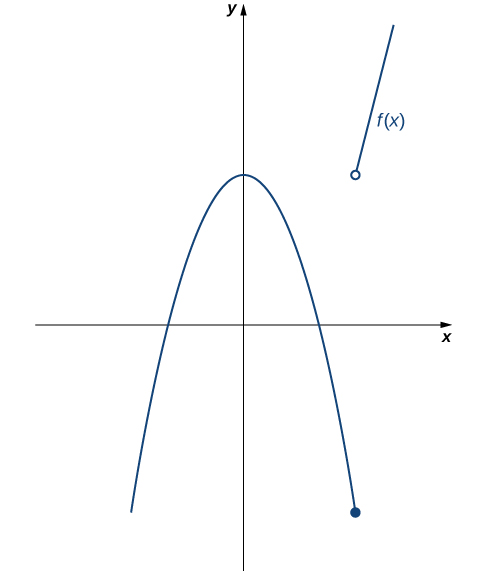3. There is an open circle at the end of the line where x would be 3.” width=”487″ height=”575″> Figure 5. The function  is not continuous at 3 because  does not exist.

By applying the definition of continuity and previously established theorems concerning the evaluation of limits, we can state the following theorem.

### Continuity of Polynomials and Rational Functions

Polynomials and rational functions are continuous at every point in their domains.

## Proof

Previously, we showed that ifandare polynomials,for every polynomialandas long as. Therefore, polynomials and rational functions are continuous on their domains.We now apply (Figure) to determine the points at which a given rational function is continuous.

# Types of Discontinuities

As we have seen in (Figure) and (Figure), discontinuities take on several different appearances. We classify the types of discontinuities we have seen thus far as removable discontinuities, infinite discontinuities, or jump discontinuities. Intuitively, a removable discontinuity is a discontinuity for which there is a hole in the graph, a jump discontinuity is a noninfinite discontinuity for which the sections of the function do not meet up, and an infinite discontinuity is a discontinuity located at a vertical asymptote. (Figure) illustrates the differences in these types of discontinuities. Although these terms provide a handy way of describing three common types of discontinuities, keep in mind that not all discontinuities fit neatly into these categories.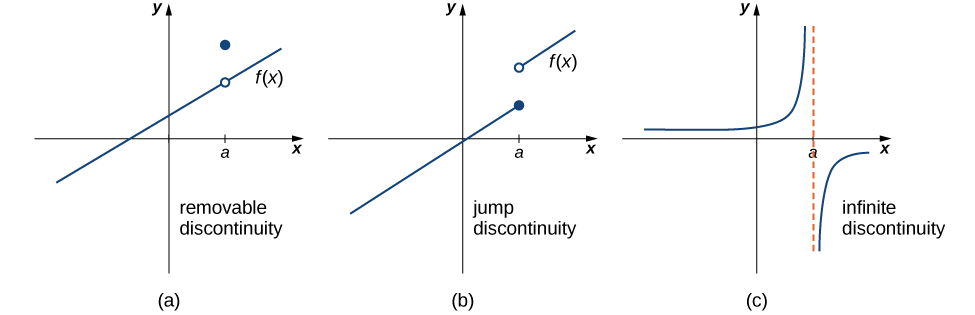0, there is an open circle on the line and a closed circle a few units above the line. The second is a jump discontinuity. Here, there are two lines with positive slope. The first line exists for x<=a, and the second exists for x>a, where a>0. The first line ends at a solid circle where x=a, and the second begins a few units up with an open circle at x=a. The third discontinuity type is infinite discontinuity. Here, the function has two parts separated by an asymptote x=a. The first segment is a curve stretching along the x axis to 0 as x goes to negative infinity and along the y axis to infinity as x goes to zero. The second segment is a curve stretching along the y axis to negative infinity as x goes to zero and along the x axis to 0 as x goes to infinity.” width=”975″ height=”315″> Figure 6. Discontinuities are classified as (a) removable, (b) jump, or (c) infinite.

These three discontinuities are formally defined as follows:

# Continuity over an Interval

Now that we have explored the concept of continuity at a point, we extend that idea to continuity over an interval. As we develop this idea for different types of intervals, it may be useful to keep in mind the intuitive idea that a function is continuous over an interval if we can use a pencil to trace the function between any two points in the interval without lifting the pencil from the paper. In preparation for defining continuity on an interval, we begin by looking at the definition of what it means for a function to be continuous from the right at a point and continuous from the left at a point.

A function is continuous over an open interval if it is continuous at every point in the interval. A functionis continuous over a closed interval of the formif it is continuous at every point inand is continuous from the right atand is continuous from the left at. Analogously, a functionis continuous over an interval of the formif it is continuous overand is continuous from the left at. Continuity over other types of intervals are defined in a similar fashion.

Requiring thatandensures that we can trace the graph of the function from the pointto the pointwithout lifting the pencil. If, for example,, we would need to lift our pencil to jump fromto the graph of the rest of the function over.

The proof of the next theorem uses the composite function theorem as well as the continuity of

and

at the point 0 to show that trigonometric functions are continuous over their entire domains.

# Proof

We begin by demonstrating thatis continuous at every real number. To do this, we must show thatfor all values of.

The proof thatis continuous at every real number is analogous. Because the remaining trigonometric functions may be expressed in terms ofandtheir continuity follows from the quotient limit law.As you can see, the composite function theorem is invaluable in demonstrating the continuity of trigonometric functions. As we continue our study of calculus, we revisit this theorem many times.

# The Intermediate Value Theorem

Functions that are continuous over intervals of the form, whereandare real numbers, exhibit many useful properties. Throughout our study of calculus, we will encounter many powerful theorems concerning such functions. The first of these theorems is the Intermediate Value Theorem.